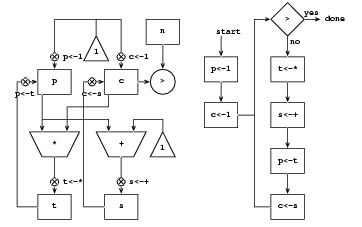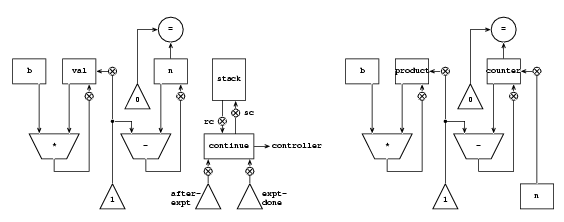\$B&K(B5.1\$B@a(B

\$BLdBj(B 5.1\$B!!(B \$BLdBj(B 5.2\$B!!(B \$BLdBj(B 5.3\$B!!(B \$BLdBj(B 5.4\$B!!(B \$BLdBj(B 5.5\$B!!(B
\$BLdBj(B 5.6\$B!!(B

\$BLdBj(B 5.1```(controller
(assign p (const 1))
(assign c (const 1))
test-c
(test (op >) (reg c) (reg n))
(branch (label factorial-done))
(assign t (op *) (reg c) (reg p))
(assign p (reg t))
(assign s (op +) (reg c) (const 1))
(assign c (reg s))
(goto (label test-c))
factorial-done)
```
\$BLdBj(B 5.3
```(controller
square-root-loop
(assing guess (const 1.0))
test
(assign a (op square) (reg x))
(assign b (op -) (reg a) (reg x))
(assing c (op abs) (reg b))
(test (op <) (reg c) (const 0.001)))
(branch (label square-root-done))
(assign d (op /) (reg x) (reg quess))
(assign e (op +) (reg guess) (reg d))
(assign guess (op /) (reg e) (const 2))
(goto (label test))
square-root-done
(perform (op print) (reg guess))
(goto (lable square-root-loop)))
```
\$BLdBj(B 5.4
```a
(controller
(assign continue (label expt-done))
expt-loop
(test (op =) (reg n) (const 0))
(branch (label return))
(save continue)
(assign continue (label after-expt))
(assign n (op -) (reg n) (const 1))
(goto (label expt-loop))
after-expt
(restore continue)
(assign val (op *) (reg b) (reg val))
(goto (reg continue))
return
(assign val (const 1))
(goto (reg continue))
expt-done)

b
(controller
(assign counter (reg n))
(assign product (const 1))
expt-loop
(test (op =) (reg counter) (const 0))
(branch (label expt-done))
(assign counter (op -) (reg counter) (const 1))
(assign product (op *) (reg b) (reg product))
(goto (label expt-loop))
expt-done)

;;\$BLdBj(B5.7\$B;2>H(B
```\$BLdBj(B 5.6
```\$B?^(B5.12 \$B!V(B;;Fib(n-2)\$B\$r7W;;\$9\$k\$h\$&@_Dj!W\$NA08e\$N(B
restore continue \$B\$H(B
save continue
```